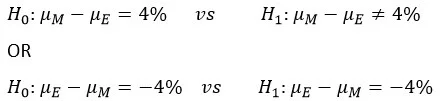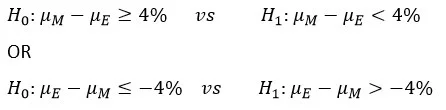06 Dec 2021

Posted on:

06 Dec 2021

1

# Z-score can be both +ve or -ve depending on E-M or M-E

While illustrating the hypothesis, you say you can go with E - M = -4 or M - = +4, but this initial assumption will determine the sign of the z score(+/-2.44). Won't this initial assumption make the final part of the lecture where we determine whether the difference is < or > than 4 confusing? Please explain in detail.

Posted on:

06 Dec 2021

0

With a belief (by the dean) that "on average, management students outperforms engineering students by 4%" can be translated asThe lecture used the second pair of hypotheses. With a two-tailed test and 5% alpha, we will reject
the null hypothesis if the z-score is less than -1.96 or if the z-score is higher than 1.96. With z-score of -2.44, we reject the null hypothesis. Now we know that the difference is not 4%.
-----------
If it is not 4%, it could be either higher than 4% or lower than 4%.
If we would like to further analyze our data and proceed with the test that the difference is higher than 4%, our hypotheses will be eitherWith this new direction of the test, the null hypothesis (with the left-tailed test) will be rejected if the z-score is less than -1.645. With the difference µM - µE, our z-score is 2.44 which is not less than -1.645. Hence, we do not reject the null hypothesis. Therefore, the average difference is not less than 4%. With the right-tailed test, we reject the null hypothesis if the z-score is higher than 1.645. Since the z-score is -2.44, we do not reject the null hypothesis. We have the same conclusion that the average difference is not lower than 4%.